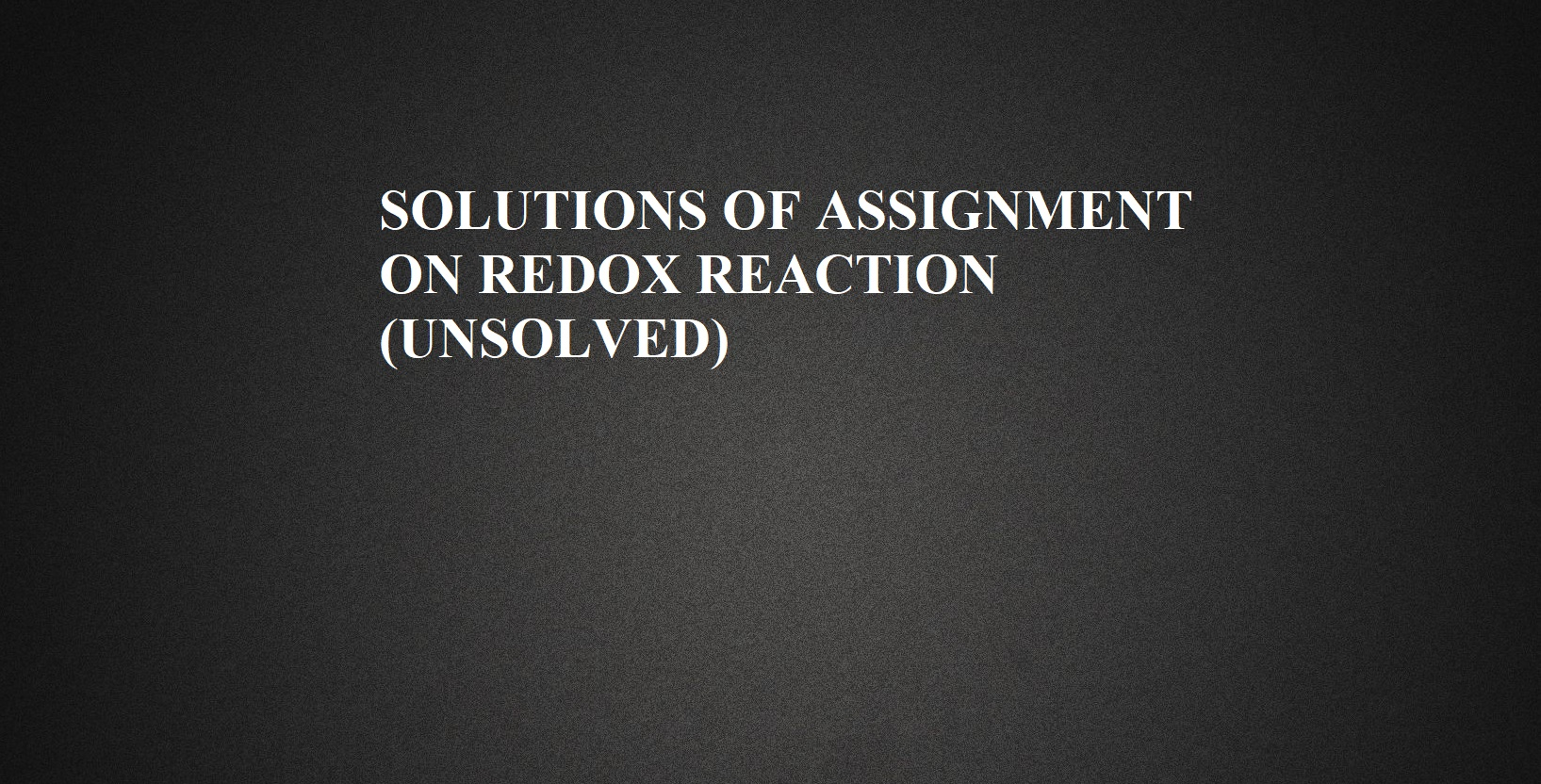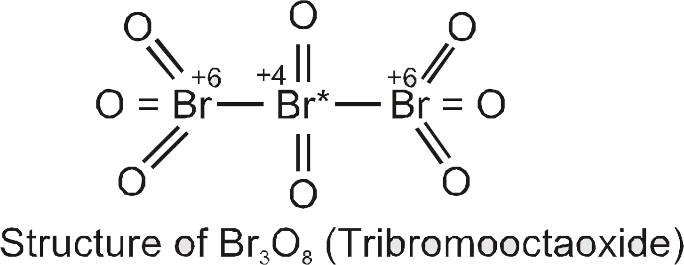# SOLUTIONS OF ASSIGNMENT ON REDOX REACTION (UNSOLVED)ASSIGNMENT ON REDOX REACTION (UNSOLVED)

# SOLUTIONS OF ASSIGNMENT ON REDOX REACTION (UNSOLVED)

SOLUTIONS OF ASSIGNMENT ON REDOX REACTION (UNSOLVED)

Ans 1.  (d) Oxidation of Cu atoms.
Reason: When copper turnings are added to silver nitrate solution, the solution becomes brown in color after some time because copper is more reactive than silver so it displaces silver from silver nitrate solution and forms copper nitrate solution.

Ans 2. (a) Increase in oxidation number
Reason: We know oxidation involves
Addition of O2 or electronegative element, Removal of H/electropositive element, Loss of electrons, Increase in oxidation number.

Ans 3. (d) KMnO4.
Reason: The electronic configuration of Mn is:
Mn (25) = [Ar]3d5 4s2, 4p0
In an excited state, it can lose all 7 electrons.
Hence, the maximum oxidation state exhibited by Mn is +7 which is in KMnO4.

Ans 4. (d) 50 mL of 0.1 M C2H2O4
Reason: 2 MnO4 + 5 C2O42- + 16 H+ → 2 Mn2+ + 10 CO2 + 8 H2O
Therefore, 2 moles of MNO4equivalent to 5 moles of C2O42-
20 mL of 0.1 M KMnO4 = 2 moles of KMnO4
Also, 50 mL of 0.1 M C2O42-equivalent to 5 mol of C2O42-
Therefore, these are equivalent.

Ans 5. (c) CrO2-4 → Cr2O72-
Reason: In VO2+ →V2​O3, V is reduced from +4 to +3 oxidation state.
In Na → Na2+, Na is oxidized from to +1 oxidation state.
In CrO4-2​ → Cr2O7-2, Cr remains in the same oxidation state +6.
In Zn+2 → Zn, Zn is reduced from +2 to 0 oxidation state.

SOLUTIONS OF ASSIGNMENT ON REDOX REACTION (UNSOLVED)

Ans 6.  (b) Two
Reason: In an alkaline medium, the reduction of KMnO4 with KI will take place as
2 KMnO4 + H2O → 2 KOH + 2 MnO2
KI + 3[O] → KIO3
Hence the overall reaction is
KI + 2KMnO4 + H2O → KIO3 + 2 KOH + 2 MnO2
So, one mole of KI will reduce two moles of KMnO4

Ans 7. (a) Formation of Fe (CO)5 from Fe.
Reason: The oxidation number of Fe in Fe(CO)5 is zero.
In both Fe and Fe(CO)5, the oxidation state of iron is zero.

Ans 8. (a) 0
Reason: CO (carbonyl) is a neutral ligand, hence oxidation state of Cr in Cr (CO)6 is zero

Ans 9. (a) Formation of slaked lime from quick lime
Reason: In this reaction
CaO + H2​O → Ca(OH)2
Oxidation number doesn’t change so it’s not a redox reaction.

Ans 10. (a) + 7
Reason: Cl shows a different oxidation state as -1 to +7 due to vacant d orbital. As oxygen is more electronegative than Cl. Oxygen size is small hence it’s more electronegative and shows -2 oxidation states.
Here Cl2O7 then equation is: 2x + 7 × (-2) = 0
x = +7 hence oxidation state of Cl is +7.

Ans 11.  (b) +6
Reason: M3+on losing 3 elections will become M+6 and O.N = + 6.

Ans 12. Na2S4O6 Let O.N.of S be x then 2 × (+ 1) + 4 ×(x) + 6 × (2) = 0
X = 2.5. By chemical bonding method the two S. atoms have O.N. + 5 and two S.

Ans 13. (c) +2
Reason: Oxidation state of oxygen in OF2 = x + (-1) × 2
= x = + 2

SOLUTIONS OF ASSIGNMENT ON REDOX REACTION (UNSOLVED)

Ans 14. (d) Zn + 2AgCN → 2Ag + Zn (CN)2
Reason: Oxidation Number of Zn changes from 0 to +2 i.e Oxidation occurs
Oxidation Number of Ag changes from +1 to 0 i.e Reduction occurs

Ans 15. (b) 2KBr + I2 →2KI + Br2
Reason: Reaction is not possible because Br ion is not oxidized in Br2, with I2, due to higher Electrode (oxidation) potential of I2 than bromine.

Ans 16. (a) The oxidation number of hydrogen is always +1.
Reason: The oxidation number of hydrogen is -1 in metal hydrides like NaH.

Ans 17. (d) 3d54s2
Reason: Highest O.N. of any transition element = (n – 1)d electrons + ns electrons. Therefore, the larger the number of electrons in the 3d orbitals, the higher is the maximum O.N.
(a) 3d14s2= 3;
(b) 3d2 4s2 = 3 + 2 = 5;
(c) 3d54s1=5 + 1=6
(d) 3d54s2 = 5+2 = 7

Ans 18. (c)
Reason: Being the most electronegative element, F can only be reduced, and hence it always shows an oxidation number of-1. Further, due to the absence of d-orbitals, it cannot be oxidized, and hence it does not show +ve oxidation numbers. In other words, F cannot be simultaneously oxidized as well as reduced and hence does not show disproportionation reactions.
Ans 19. (b) Formation of H2O2 by the oxidation of H2O.
Reason: Autoxidation is the spontaneous oxidation of a compound in air.
Autoxidation produces hydroperoxides and cyclic organic peroxides. These species can react further to form many products. The process is relevant to many phenomena including aging, paint, and spoilage of foods, degradation of petrochemicals, and the industrial production of chemicals. Autoxidation is important because it is a useful reaction for converting compounds to oxygenated derivatives, and also because it occurs in situations where it is not desired (as in the destructive cracking of the rubber in automobile tires or in rancidification). Water automatically gets oxidized to hydrogen peroxide.

SOLUTIONS OF ASSIGNMENT ON REDOX REACTION (UNSOLVED)

Ans 20. (c) Oxidation Potential
Reason: The magnitude of the electrode potential of a metal is a measure of its relative tendency to lose or gain electrons. i.e., it is a measure of the relative tendency to undergo oxidation (loss of electrons) or reduction (gain of electrons).
M → Mn+ + ne– (oxidation potential)
Mn+ + ne– → M (reduction potential)

Ans 21. (c) Equal to Number of moles of electrons Lost or gained by one mole of reductant or oxidant
Reason: For redox reaction, it is considered a change in their oxidation number on both sides of a chemical reaction.

Ans 22. (d) + 5, + 6 and + 6
Reason: P in  PO43–
X + 4 (-2) = -3 = X – 8 = -3
X =     -3 + 8 = + 5
S in SO42–
X + 4(-2) = -2 = X – 8 = -2
X = -2 + 8 = + 6
Cr in Cr2O72–
2X + 7 (-2) = -2
2X – 14 = -2
2X = -2 + 14 = 12
X = + 6

Ans 23. (d) 8/3
Reason: 3X + 4(-2) + 0
3X – 8 = 0
X = 8/3

Ans 24. (a) CO2
Reason: Reducing agent means to reduce other and to oxidize themselves. Therefore, carbon cannot show an oxidation state of more than +4.

Ans 25. (a) Positive
Reason: Any redox reaction would occur spontaneously if the free energy change (ΔG) is negative.
ΔG0 = – nFE0
Where n is the number of electrons involved, F is the value of faraday, and E0 is the cell emf. ΔG0 can be negative if E0 is positive.

SOLUTIONS OF ASSIGNMENT ON REDOX REACTION (UNSOLVED)

ASSERTION REASONING BASED QUESTIONS

Ans 26. (B), Both assertion and reason are true but the reason is not the correct explanation of the assertion.
Ans 27. (D), The assertion and reason both are false.
Ans 28. (B), Both assertion and reason are true but the reason is not the correct explanation of the assertion.
Ans 29. (A), Both assertion and reason are true and the reason is the correct explanation of the assertion.
Ans 30. (C), The assertion is true but the reason is false.
Ans 31. (B), Both assertion and reason are true but the reason is not the correct explanation of the assertion.
Ans 32. (A), Both assertion and reason are true and the reason is the correct explanation of the assertion
Ans 33. (D), The assertion is false but the reason is true.
Ans 34. (A), Both assertion and reason are true and the reason is the correct explanation of the assertion
Ans 35. (A), Both assertion and reason are true and the reason is the correct explanation of the assertion
Ans 36. (C). The assertion is true but the reason is false.
Ans 37. (E). If the assertion and reason both are false.
Ans 38. (A), Both assertion and reason are true and the reason is the correct explanation of the assertion
Ans 39. (B), Both assertion and reason are true but the reason is not the correct explanation of the assertion
Ans 40. (C). The assertion is true but the reason is false.

SOLUTIONS OF ASSIGNMENT ON REDOX REACTION (UNSOLVED)

COMPREHENSION BASED QUESTIONS

Ans 41. The oxidation number of Br in Br3O8 has structural dependence.Each of the two-terminal Bromine atoms has 3 oxygen atoms attached to it. So, the bromine atom on the terminals will have an oxidation state +6. The bromine atom in the middle has 2 oxygen atoms attached to it. It will have an oxidation state of +4.

Ans 42. The reaction taking place is
Cu → Cu2+ + 2e

Ans 43. + 6. Due to the presence of peroxide linkage.

Ans 44. 2Cu+ → Cu2+ + Cu

Ans 45. 3MnO42– + 4H+ → 2MnO4 + MnO2 + 2H2O

SOLUTIONS OF ASSIGNMENT ON REDOX REACTION (UNSOLVED)

ASSIGNMENT ON REDOX REACTION (UNSOLVED)